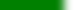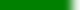The following is simply a conveniently formatted list of learning goals. More likely than not, some might be hard to assess through a quiz or exam. Students should prioritize their studying based on:

• how much time in lecture was spent discussing or understanding the topic,
• how much the topic was stressed in learning group assignments
• and the student's comfort level with the topic

# Assessable Learning Goals covered for Quiz 1Know the rules for presenting discrete and continuous data relationships (scatter plots vs connected dots).Know how to write Monte Carlo simulations for estimating the Pr(A) of an event A.What are replications in the context of discrete event simulations?What (arithmetic) pRNG properties did the thieves take advantage of in electronic poker games?Explain the values a, m, g() and x0 in a (Lehmer) PMMLCG; how is the next Uniform(0,1) random value calculated?What does the pRNG API routine Random() provide to a simulation writer?What is a full period multiplier and why is it important to Lehmer pRNGs?Explain what "random numbers fall mainly in the planes" means.What is the geometric interpretation of multiple Lehmer pRNG streams?Know how to calculate traffic intensity and its connection to service rate.Understand how a FIFO SSQ simulation can be written in a simple while loop and how a_i and s_i can be manipulated for simple experiments.Understand the canonical SSQ and appreciate its broad application to computer simulation.Know the Equilikely(a,b) random variate: the meaning of its parameters, pmf, and CDF.Know the Uniform(a,b) random variate: the meaning of its parameters, pdf, and CDF.What does the parameter u in random variates represent? Computationally, how do we get a value for u in code?